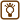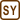What is the total electrical resistance of the following circuits if all the resistors have the same resistance R?

a)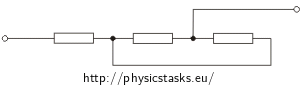b)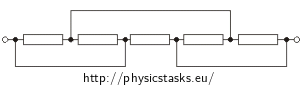c)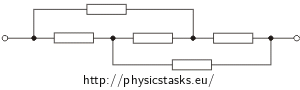• #### Hint 1

The key to a successful solving of the problem is to draw the circuit in a more familiar and common way so that it is clear if it is in series or parallel.

• #### Hint 2

"Bending" of wires during drawing the networks does not change any properties of network neither total resistance of the network.

Resistors with no current flowing through them (including ones that are short-circuit) can be left out.

• #### Breakdown

During computation of total resistance it is necessary to have uncluttered schematic of the circuit. There are no general instructions for making that schematic. However, there are few tricks you can often use:

1. Wires in integration can be bend, shortened and lengthened without affecting the total resistance of the integration. According to this the following two images show the same wiring: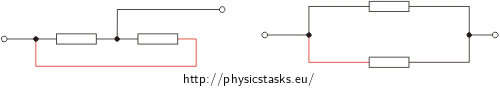2. If a wire connects two knots and there is no appliance on that wire we can merge these knots into one. Conversely, it is possible to divide one knot into two connected by wire on which there is no resistor. For example, the following two pictures show the same wiring: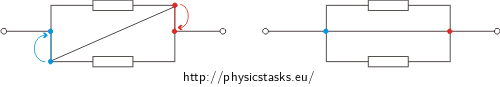3. Resistors through which there is no flowing current can be taken out of the circuit without changing its properties. For example, in the left picture the current if flowing through the middle branch in the parallel part of circuit because there is no resistor, and so there is zero resistance. The other two branches are short-circuited so they do not affect the total resistance and can be left out of the image. The picture shows the two same circuits: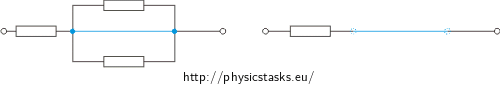We will be solving the rest of the circuits using similar ideas.

During computation of total resistance we will use following rules:

1. For combination of resistors in series the total resistance is equal to the sum of resistances of each resistor.
2. For combination of resistors in parallel the reciprocal value of total resistance is equal to sum of reciprocal values of resistance of each resistor.
• #### Solution of part a)

"Bending" and change of length of wire during redrawing of a schematic does not affect any properties of the circuit including total resistance.

In the picture we marked wires that are going to be bent blue.

Now we can start computing the total resistance as a sum of one resistor R and resistance of the parallel part of the circuit.

Parallel part of the circuit consists of 2 resistors in parallel. For resistance Rp of this part we can write:

$\frac{1}{R_p}\,=\,\frac{1}{R}\,+\,\frac{1}{R}$ $R_p\,=\,\frac{R}{2}\,.$

Total resistance of the circuit is:

$R_\mathrm{total}\,=\,R_p\,+\,R\,=\,\frac{R}{2}\,+\,R$ $R_\mathrm{total}\,=\,\frac{3R}{2}\,.$
• #### Solution of part b)

First of all we will mark the knots which are connected with wires without any resistors using colours. Knots and wires with same colour are basically just one knot. We will also mark flowing of current through each knot.

Now we will again redraw the circuit where we will "bend" the coloured wires. Easy way to check if the redrawn schematic is correct is to check the currents in the knots.

Now when we have redrawn it in a more comprehensive way, we can compute total resistance.

Circuit consists of two parallel branches. In the upper branch there are two parts connected together. In each of these two parts there are two resistors in parallel. We can compute the resistance Rp this way:

$\frac{1}{R_p}\,=\,\frac{1}{R}\,+\,\frac{1}{R}\,=\,\frac{2}{R}$ $R_p\,=\,\frac{R}{2}\,.$

Resistance of the upper branch is:

$R_u\,=\,2R_p\,=\,R .\tag{upper branch}$

Resistance of the lower branch is equal to the resistance of one resistor R:

$R_l\,=\,R .\tag{lower branch}$

For total resistance we can write:

$\frac{1}{R_\mathrm{total}}\,=\,\frac{1}{R_u}\,+\,\frac{1}{R_l}\,=\,\frac{1}{R}\,+\,\frac{1}{R}\,=\,\frac{2}{R}$ $R_\mathrm{total}\,=\,\frac{R}{2}\,.$
• #### Solution of part c)

First we will mark the knots with different colours each.

We may notice that between every 2 knots there is one resistor (except for blue and purple knot). We will use this fact when we check if the redrawn schematic is correct.

Now we will redraw the integration using "bending" and "shortening" of the wires.

With this circuit it is not so easy to compute the total resistance even after the redrawing. Now we will look closely at the scheme one more time and make it even simpler.

Here are a couple of observations:

1. Current that enters into the blue knot from the left must leave from the purple knot.

2. Circuit is symmetrical.

3. From the symmetry we can see that the current flowing through blue knot is divided into two equal parts into the upper and lower branch.

4. 4. These two currents merge back into one at the purple knot.

From point 3 and 4 we can say that in between green and orange knot there is no current flowing. Therefore we can take the resistor between these knots out without changing the total resistance.

Now we can easily compute the total resistance of the integration. First we will determine the resistance of upper branch Ru and then Rl. We can say:

$R_u\,=\,R_l\,=\,R\,+\,R\,=\,2R\,.$

Total resistance of the circuit is:

$\frac{1}{R_\mathrm{total}}\,=\,\frac{1}{R_u}\,+\,\frac{1}{R_l}\,=\,\frac{1}{2R}\,+\,\frac{1}{2R}\,=\,\frac{1}{R}$ $R_\mathrm{total}\,=\,R\,.$

Comment: Total resistance can be also determined with Kirchhoff laws. You will find this method of solving properly commented here Application of Kirchhoff’s laws for calculation of total resistance in a circuit.

Total resistances of individual circuits are: a) $$\frac{3R}{2}$$, b) $$\frac{R}{2}$$, c) $$R$$.